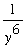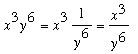SEARCH HOMEMath Central Quandaries & QueriesQuestion from Bill, a student: I need help completing this negative exponents problem: x to the power of 3 multiplied by y to the power of negative 6. What would the process to solving this problem be?Hi Bill,

y-6 is an alternate way of expressingand hencePennyMath Central is supported by the University of Regina and The Pacific Institute for the Mathematical Sciences.